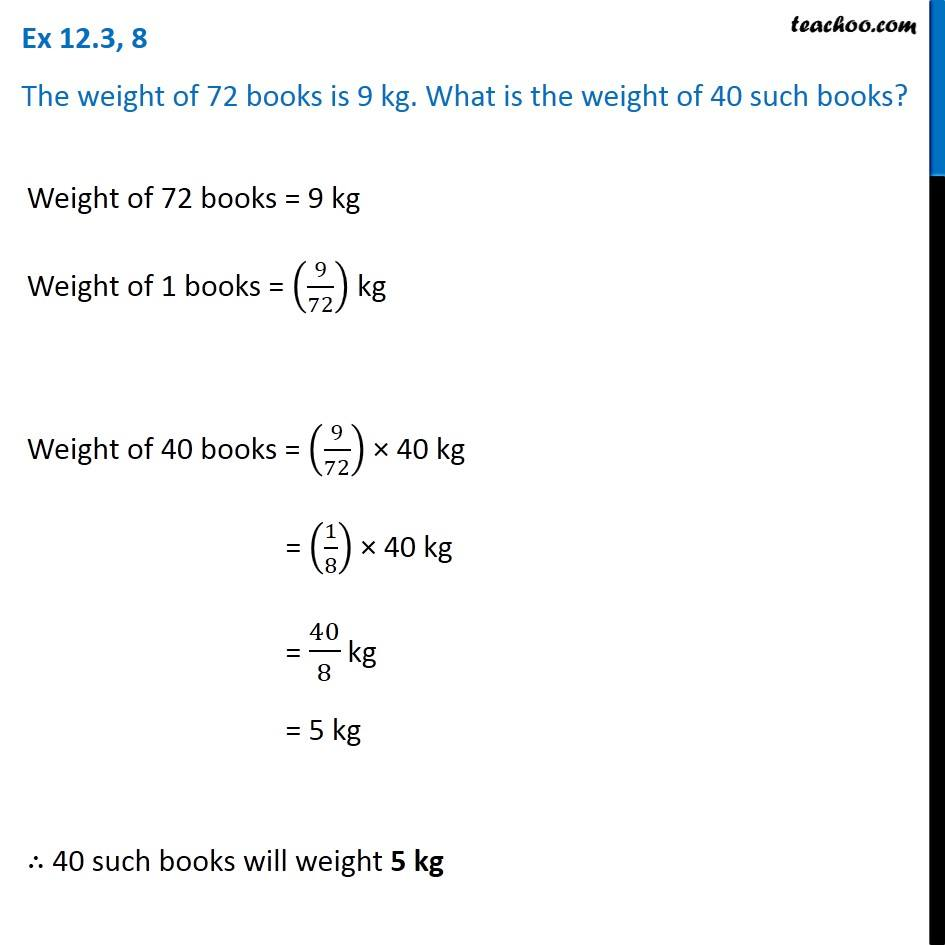Unitary Method

Chapter 12 Class 6 Ratio And Proportion
Concept wiseIntroducing your new favourite teacher - Teachoo Black, at only ₹83 per month

### Transcript

Ex 12.3, 8 The weight of 72 books is 9 kg. What is the weight of 40 such books?Weight of 72 books = 9 kg Weight of 1 books = (9/72) kg Weight of 40 books = (9/72) × 40 kg = (1/8) × 40 kg = 40/8 kg = 5 kg ∴ 40 such books will weight 5 kg